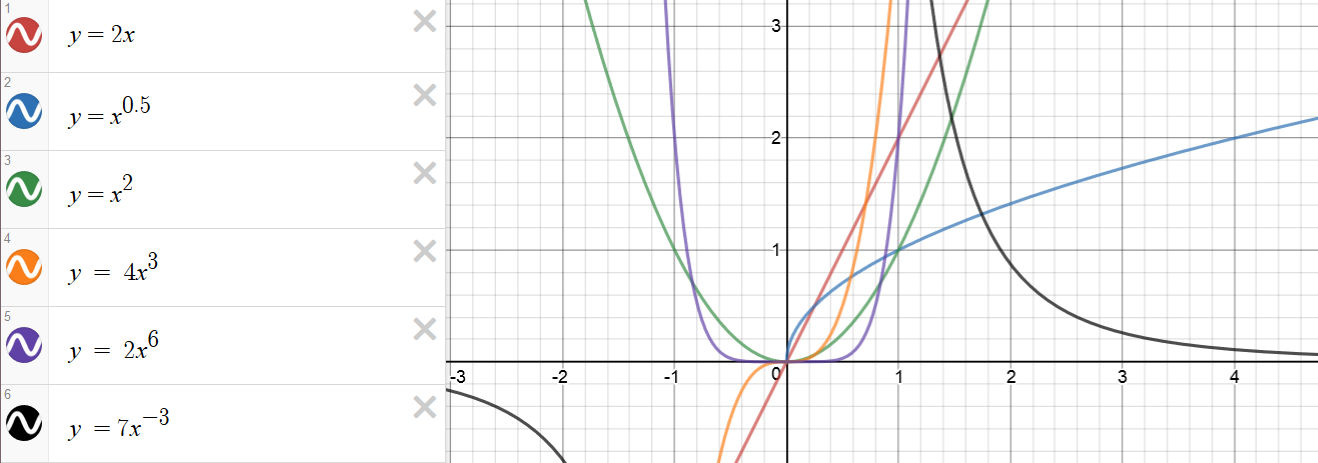# Definition of Power FunctionA power function is equal to a constant times a power of $x$.

Some examples of power functions include $y = x^3$, $y = 5x^4$, $y = 3x^9$ and $y = 5x^{-2}$.

Some other examples of power functions are shown on the graph.

### Description

The aim of this dictionary is to provide definitions to common mathematical terms. Students learn a new math skill every week at school, sometimes just before they start a new skill, if they want to look at what a specific term means, this is where this dictionary will become handy and a go-to guide for a student.

### Audience

Year 1 to Year 12 students

### Learning Objectives

Learn common math terms starting with letter P

Author: Subject Coach
You must be logged in as Student to ask a Question.